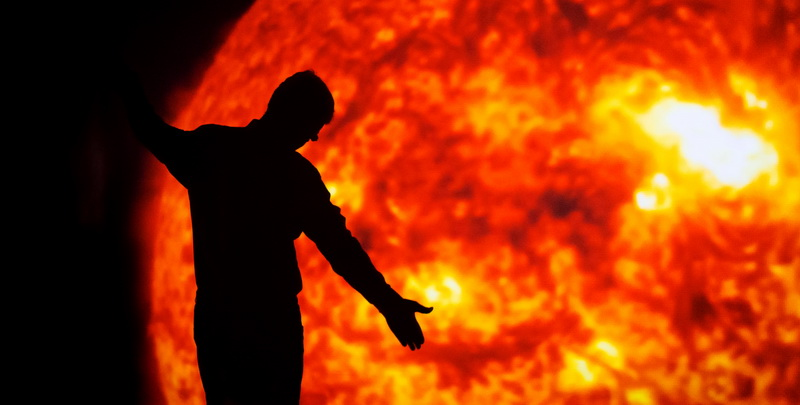© 2016 dimitri## 2017 Revolutions

2017 : Another terrestrial revolution around the sun that will vibrate our perceptions of space and time in this galactic wonder. We are suspended on a sphere of matter in an elusive immensity while playing the game of civilizations….

New year, new agitations in the matrix of our postures in this world. No war, no act of greed, no human or animal exploitation will put us down. Our dances will make us perceive, contemplate and act, in order to renew our relationship with the manifestations of life. Do you feel like sharing a moment of silence to (re-)appreciate our existence on this planet?

(…………………………………………………………………)

Newtopia 4-2, at the beginning of December, was our collective attempt to re-value silence, to slow down the careless manipulation of nature and get more access to…the present moment : invent new worlds, new nuances of touch, new texts and dances that bring us closer to the essentials of being sensitive body-souls and of living together. An experience full of images, feelings, dances and texts that amazed us with unique artistic proposals. A big thank you to all our partners for their support! See more info on Newtopia 4.2 : HERE.
Photos by by Bernard Duret
: Flickr Album Newtopia 4.2

<<<<<<<<<<<<<<<<<<<<<<<<<<<<<<<<<<<<<<<<<<<<<<<<<<<

### Artistic news :

First appointement for 2017 : Newtopia 4.3, on january 12th, at the Bretonneau University Hospital of Tours. A choreographic meeting with members of the Omnivion association dedicated to the hospital public.

<<<<<<<<<<<<<<<<<<<<<<<<<<<<<<<<<<<<<<<<<<<<<<<<<<<<

In this month of January we’ll have the first rehearsals of Newtopia 4.4 : two choreographic creations by Dimitri Tsiapkinis, scheduled for May 17th at the Pléiade, in La Riche (France, 37520). Moreover, Dimitri will be on tour with O.More in Brittany. O.More is a legendary piece on Shakespeare’s Othello by Bernardo Montet, that has been on tour since 2002 in Europe and Africa. Info : ciemawguerite.com

<<<<<<<<<<<<<<<<<<<<<<<<<<<<<<<<<<<<<<<<<<<<<<<<<<<<<

And in February, we’ll begin the rehearsals for “Orange Bear Lights II” (F.O.D.O. II), a one-man-show by Dimitri Tsiapkinis, for the 2017-2018 season. Info : omnivion.net/fodo_II

<<<<<<<<<<<<<<<<<<<<<<<<<<<<<<<<<<<<<<<<<<<<<<<<<<<<<

Arts Education news :

• Restart of the Wednesdays’ dance workshops on January 4th at the Rabière. There are very few places left for new participants. Here are some pictures : flickr album ateliers 2016
• The workshop Lapis-Lazuli, dance for teenagers at the psychiatric center of the University Hospital of St.Cyr-sur-Loire continues on January 6. Info : lapis-lazuli
• The Indigo workshops  / dance and mental health for adults will begin on january 10th at the University Hospital of Tours. Info : Indigo
• The dance workshop series with Dimitri Tsiapkinis, for amateurs at the National Choreographic Center of Orléans, is scheduled for January 7th. Photos : kr/CCNO

<<<<<<<<<<<<<<<<<<<<<<<<<<<<<<<<<<<<<<<<<<<<<<<<<<<<<

Memo on subscribing to Omnivion :

From now on, our members can pay their subscription through the e-cotiz micro-site by credit card : e-cotiz.com/omnivion
Your support is precious! As the service costs us 1 € per membership, it’s up to you if you offer us that extra euro and pay 6€ instead of 5€!
Photographic albums testify of our dance adventures for the past 5 years: flickr Omnivion albums (2011-2016)
Keep in touch here, or on our Facebook page for more info.

<<<<<<<<<<<<<<<<<<<<<<<<<<<<<<<<<<<<<<<<<<<<<<<<<<<<<<<

Dimitri Tsiapkinis and the whole Omnivion team warmheartedly greet you!

<<<<<<<<<<<<<<<<<<<<<<<<<<<<<<<<<<<<<<<<<<<<<<<<<<<<<<<<<<<